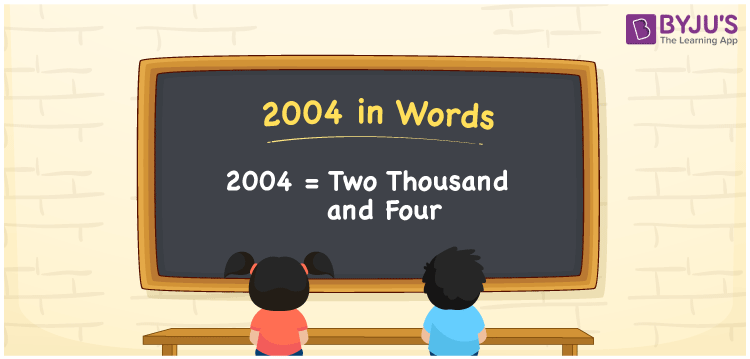# 2004 in Words

2004 in words can be written as Two Thousand and Four. For example if you buy a saree for Rs. 2004, then you can say that “I bought a saree for Two Thousand and Four Rupees”. The English alphabet is made to write 2004 in words. The step wise explanation given for writing numbers in words provides a strong conceptual base among the students. In English, 2004 can be read as “Two Thousand and Four”.

 2004 in words Two Thousand and Four Two Thousand and Four in Numbers 2004

## 2004 in English Words## How to Write 2004 in Words?

In this section, you will learn more about the concept of writing 2004 in words. There are four digits in the number 2004. Below you will find the place value chart which shows the place value of all the four digits.

 Thousands Hundreds Tens Ones 2 0 0 4

The expanded form of the number 2004 is provided below:

2 x Thousand + 0 × Hundred + 0 × Ten + 4 × One

= 2 x 1000 + 0 × 100 + 0 × 10 + 4 × 1

= 2000 + 4

= 2004

= Two Thousand and Four

Therefore, 2004 in words is written as Two Thousand and Four.

2004 is a natural number that precedes 2005 and succeeds 2003.

2004 in words – Two Thousand and Four

Is 2004 an odd number? – No

Is 2004 an even number? – Yes

Is 2004 a perfect square number? – No

Is 2004 a perfect cube number? – No

Is 2004 a prime number? – No

Is 2004 a composite number? – Yes

## Frequently Asked Questions on 2004 in Words

Q1

### Write 2004 in words.

2004 in words is Two Thousand and Four.
Q2

### What is the value of 2010 minus 6?

The value of 2010 minus 6 is 2004. Therefore, 2004 in words is Two Thousand and Four.
Q3

### Is 2004 a perfect cube?

No, 2004 is not a perfect cube as it cannot be expressed as the product of three integers which are equal.